Next: The cross section Up: The physical processes producing Previous: The physical processes producing   Contents

## Proton-Proton collisions

In collisions between high energy protons and nuclei, pions of all charges,,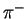and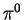are produced as secondaries.andgenerate electrons and positrons, while the neutraldecades in a pair of gamma photons. The energy of the emitted gamma is, in the reference frame of the, half of therest mass, and therefore the observed energy of the gamma ray depends on the momentum ofrespect to the observer.Ifis the spectra of protons(1.8)

theemissivity per hydrogen atom is :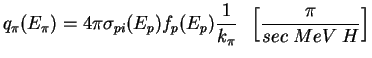(1.9)

whereis the energy of the emitted pion,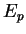is the energy of the proton,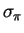is the cross section forproduction through proton-proton collision (see par. 1.5.1).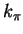is the mean fraction of proton kinetic energy transferred to the neutral pion, and is about 0.17 [Mori, 1997].andthen are related by:(1.10)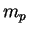is the proton rest mass. The gamma-ray emissivity results to be: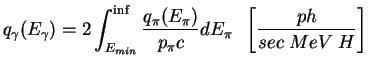(1.11)

whereis the photon observed energy,is the pion momentum and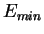is the minimal energy necessary for a pion to create a photon of energy(in the observer reference system):(1.12)

whereis the neutral pion rest mass. The emissivity per unit volume of interstellar matter is then:(1.13)

whereis the density of H atom (both atomic and molecular).

SubsectionsNext: The cross section Up: The physical processes producing Previous: The physical processes producing   Contents
Andrea Giuliani 2005-01-21